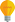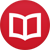# Are scientific theories objective?

by Jakub MarianTip: See my list of theMost Common Mistakes in English. It will teach you how to avoid mis­takes with com­mas, pre­pos­i­tions, ir­reg­u­lar verbs, and much more.

Virtually all physicists praise the scientific method as the best possible approach to discovering the laws of nature because it is objective. At least in theory, it sounds bullet-proof:

1) Based on experience, calculations, preceding experiments, holy scriptures, or any other sources of inspiration, create a theory (or just a single prediction) in such a way that it can be independently tested. For example “seeing red makes people feel strange” is not a scientific theory, because there is no definition of “feeling strange” that would be universally accepted and applicable to everyone.

On the other hand, “seeing red causes people to blink more often” essentially is a scientific theory because you can take anyone who is capable of seeing colours, show him something red, and measure how often they blink. It would need some clarification, such as what exactly you mean by “seeing”, “red” and “more often”, but in essence, this theory is “objectively” testable.

2) Devise an experiment to test the theory. For example in the case of red and blinking, you could make a group of people look at a neutral image while another group would be looking at an image that is only red and measure their rate of blinking. Then you should exchange the groups and perform the test again (because it may happen that one of the groups blink faster on average than the other).

3) Statistically process the data and interpret the results.

The point is that you test your theories. It’s all right if you create a theory that Earth is less than 10,000 years old based on the Bible, but the most important step in the scientific method is that you will eventually abandon the theory as experiments based on geology, paleontology, chemistry, oceanography, cosmology, etc., all provide data that are incompatible with it.

## The problem of language and interpretation

Even 1) and 2) already suffer from some problems. The first apparent problem is that of interpretation. To devise an experiment, you first have to be able to say what the theory means. While this is “obvious” for problems such as blinking, the same task can become highly nontrivial for theories formulated using abstract mathematics. As a result, interpretation becomes part of the theory.

Interpretation is something physicists agree upon, and thus it is not objective. If an experiment fails, it can either mean that the mathematical theory behind it is flawed (i.e. the experiment falsifies the theory) or that the way we interpret the theory is wrong, although the theory itself is essentially correct. For example, if you try to test the classical formula F = ma in conditions where it holds with high precision, but you interpret m as the mass of the body exerting the force instead of the mass of the body to which the force is applied, the experiment will likely fail, but it will not disprove the formula.

## There is no objective language

The need for interpretation undermines the sense of absolute objectiveness of scientific theories with which they are often presented, but generally causes little difficulty in practice. There is, however, a greater and more subtle problem: the language. Imagine the poor creature from my article about natural numbers being just a construct of our mind which is not capable of perceiving discrete objects. It would be impossible for you to explain any mathematical theory to it because our mathematics is based on discrete objects (natural numbers and symbols).

Nevertheless, since we can in a way simulate its understanding of the physical world (we can construct “fuzzy” objects in our mathematics, after all) we should be able to reformulate our theories in a language it would be able to understand. These theories would necessarily contain less information than the original, and so we would conclude that the creature’s understanding of the real world is severely limited because the principles of its cognition (and therefore of the language it uses to formulate theories) don’t allow it to understand certain phenomena.

And hence you see the catch: what if we are in fact in a situation similar to that creature? What if principles of our own cognition and discrete language don’t allow us to describe and fully understand certain phenomena (such as randomness in quantum mechanics)? And what if there is a being whose cognition works so differently from our own that it is able to actually perceive quantum effects?

Perhaps we wouldn’t even be able to testify the existence of such a being, because anything it would do would appear random to us. Nevertheless, its understanding of the universe would be far better than our own, but it would be impossible in principle to translate its theories into our mathematical language.

In other words, there may be several subjects with different types of cognition and fundamentally different languages having their own theories which are in principle untranslatable into each other’s language. Such subject-dependent theories can hardly be called objective.

## Is statistics right?

It may well happen that our theories aren’t objective in any sense, but can we be at least sure that the method we use works at least for us, humans? There’s an interesting vicious cycle of physics and mathematics: we use physical experiments in order to verify that mathematical theories we have created correspond to the real world, but we use a purely mathematical theory to eventually decide whether this indeed is the casestatistics!

Say, you have finished an experiment verifying a very abstract theory in particle physics. You statistically process the data and then say that, with a certain likelihood, the experiment falsifies the theory. As people repeat the experiment, the likelihood that the theory is wrong approaches 100%. But what if the mathematical theory you used to process the data, i.e. statistics, is itself wrong?

Statistics is also a theory about the physical world in that you interpret the numbers you read on a computer screen as certain variables within the theory. What if it happens (and this seems especially plausible for highly abstract theories) that when an experiment falsifies a theory, it is in fact the mathematical method used to process the data that is wrong, not the theory itself? The experiment would in fact falsify the theory of statistics, not the theory in question, but no-one would notice; everyone would try to find a glitch in the theory itself, not in the statistical method.

To conclude our discussion, let’s just note that statistics became a part of the formulation of the scientific method. We simply assume that it is correct when applied to data from any possible experiment, but it may happen that we will reject a correct scientific theory because the very principles of the scientific method are wrong.

## But in pure mathematics…

So it seems that we cannot really be sure about objectiveness or even (in)correctness of scientific theories. But we can be at least sure about “inner correctness” of mathematical theories? We prove theorems, and proofs are correct or incorrect whether we believe in them or not, right? Well, not really.

By the way, I have written several educational ebooks. If you get a copy, you can learn new things and support this website at the same time—why don’t you check them out?